# DAYLIGHT PREDICTOR

## INTRODUCTION

Simulating daylight isn’t as easy and accesible for everyone as it solve be. To make it easier to know, we decided to predict it using Machine Learning. The goal then is to predict the daylight autonomy (DA) in a room considering different room and window sizes. The daylight autonomy describes the percentage of occupied hours where the target daylight illuminance (typically 300 lux) is met by a sensor point.We first created our dataset, from 3000 samples, we then trained the model using some of the features of that dataset as inputs and then we tested how the prediction worked.## DATASET GENERATION

To predict daylight we defined some features in the room and window which would be the inputs for then getting the DA prediction as an output. This DA prediction would be a value from 0-100 for each of the 25 cells we divided the rooms in the 5×5 grid. These are the features we used: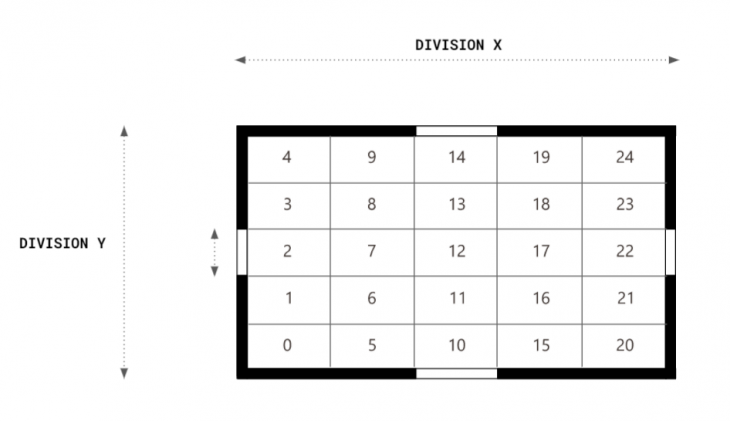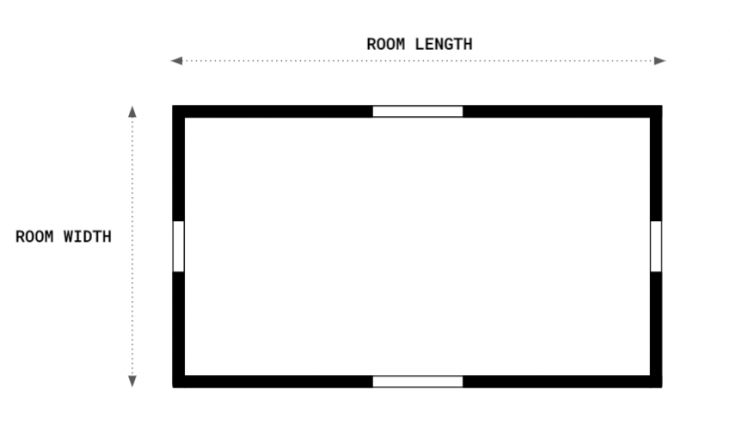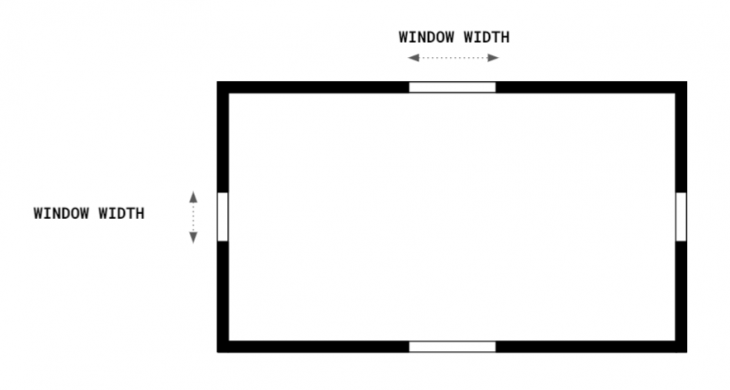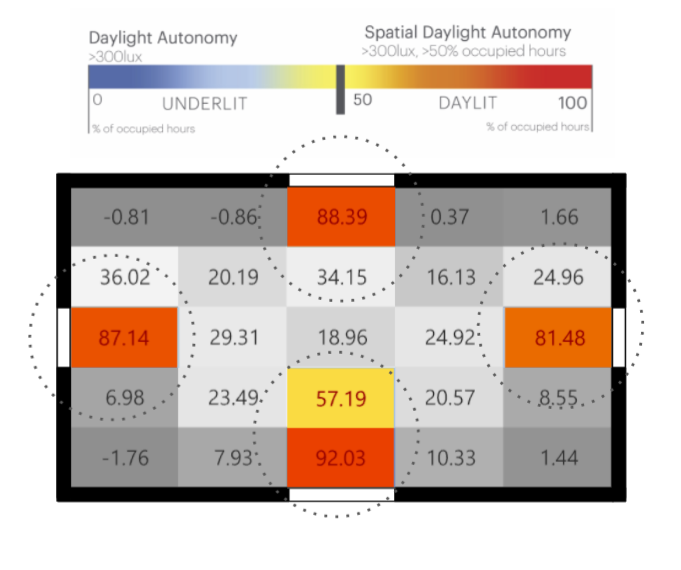By varying these parameters, we obtained our dataset made from 3000 samples.## DATASET ANALYSIS

Considering the explained features, we generated our first dataset which followed a uniform distribution. After we analyzed it we saw that we could get some bias because we had more samples which had low and high spatial daylight autonomy but they weren’t so much on the middle, so we generated a new dataset with a gaussian distribution. Finally, we added more features to that one, because we weren’t getting so much good predictions and we concluded that we needed more inputs.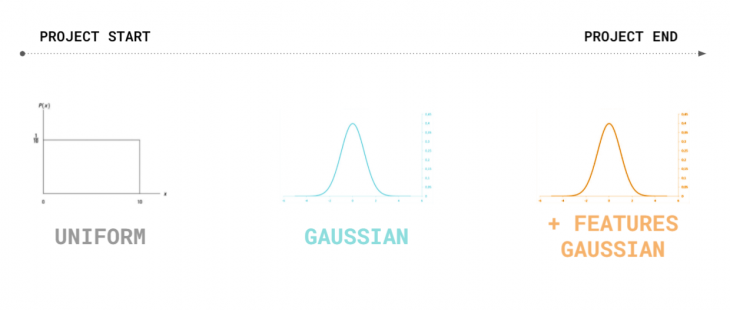## TRAINING

So, with each of these datasets, we used different features as inputs and outputs in our models which were built as an ANN using regression.

FEATURES FOR UNIFORM AND GAUSSIAN DATASETS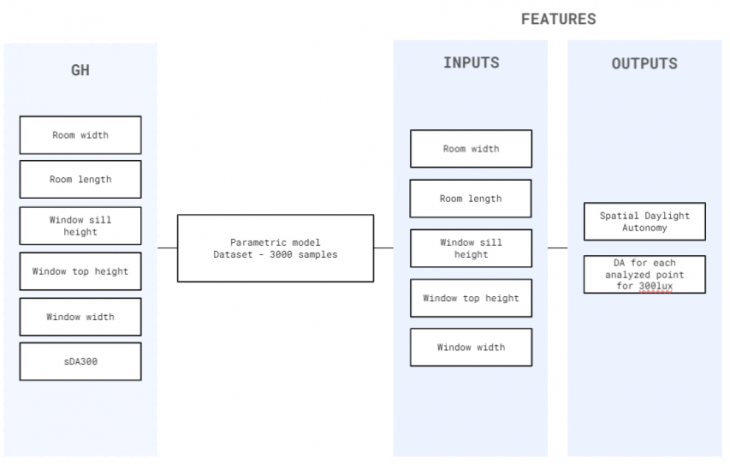FEATURES FOR +FEATURES GAUSSIAN DATASETWe also tried different model parameters to obtain the best prediction as possible, and compared them to get conclusions from them.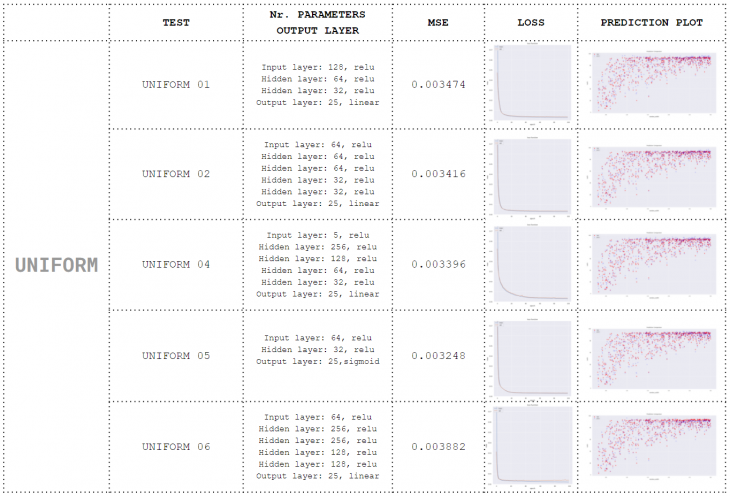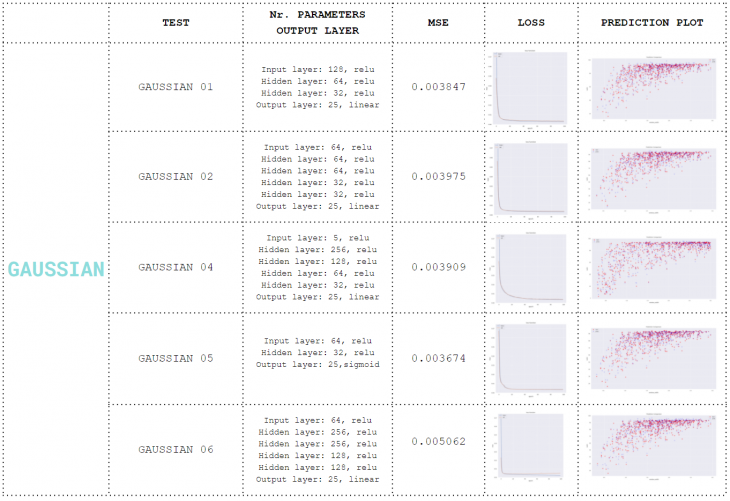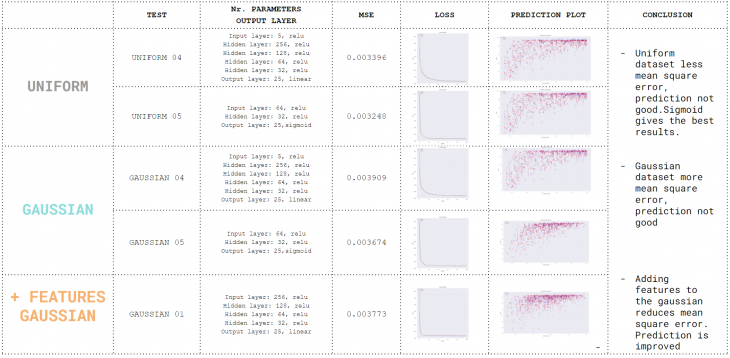## PREDICTION

The final model we used was created from the last dataset we generated. In this one we used two models. In the first one we predicted the spatial Daylight Autonomy value, which we then introduced as an input for our second model, the one that was predicting the daylight autonomy in each of the cells.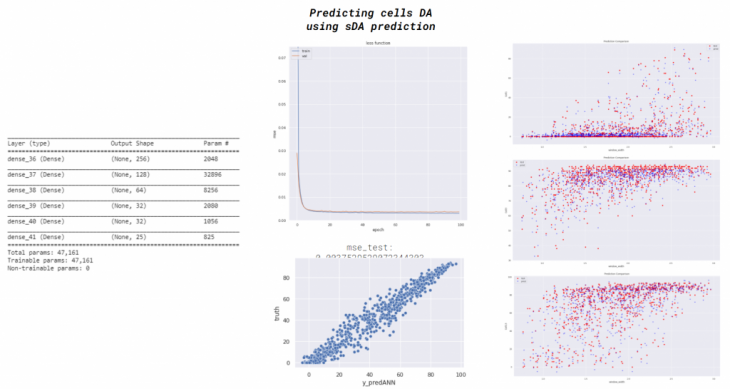After we built our model, we used hops to automatically generate the prediction using grasshopper, out from the values of the sliders. By doing so, we could also compare the prediction with the simulation we were getting from Ladybug and Honeybee.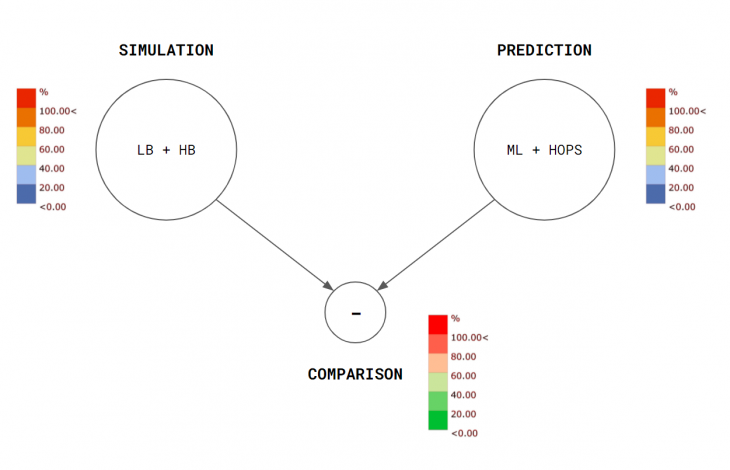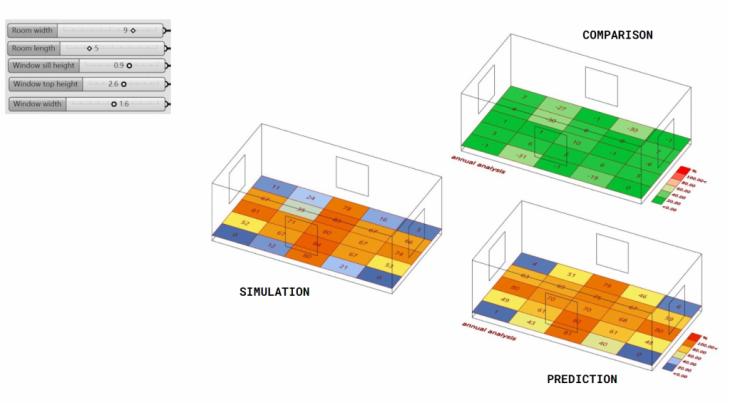DAYLIGHT PREDICTOR is a project of IAAC, Institute for Advanced Architecture of Catalonia developed at Master in Advanced Computation for Architecture & Design in 2020/21 by

Students: Jonathan Hernández López and Jaime Cordero Cerrillo

Faculty: Gabriella Rossi and Iliana Papadopoulou